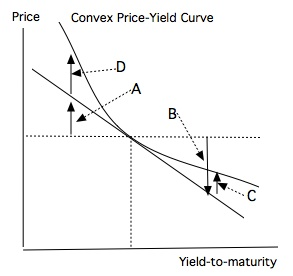### CFA Practice Question

There are 764 practice questions for this topic.

### CFA Practice Question

Refer to the following convex price-yield curve.Increasing the yield-to-maturity will cause the price to drop by ______.
A. B
B. C
C. B - C
Explanation: B is the estimated price drop due to duration and C is the estimated price increase due to convexity. B - C is the total impact.